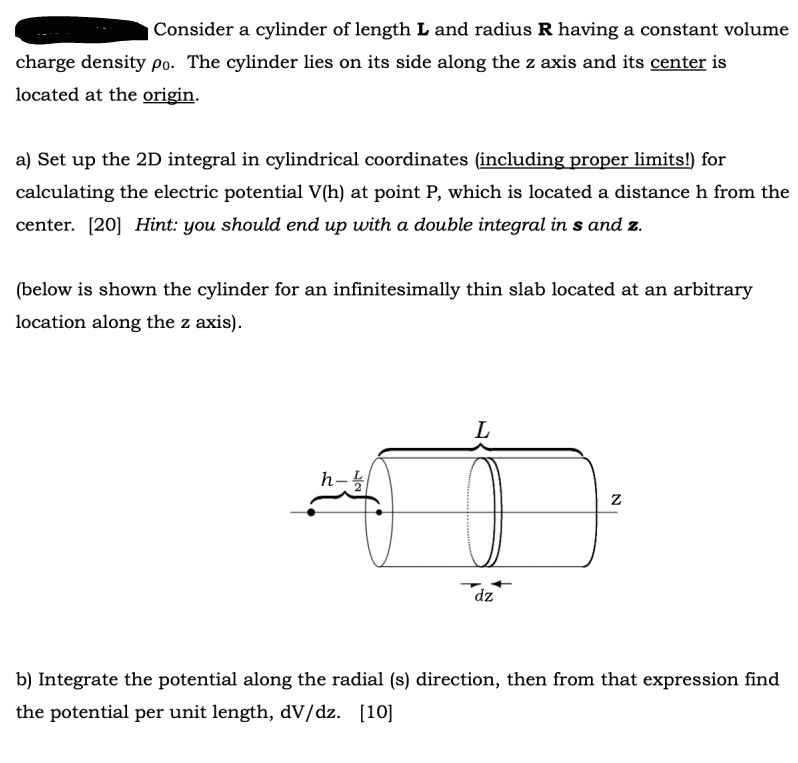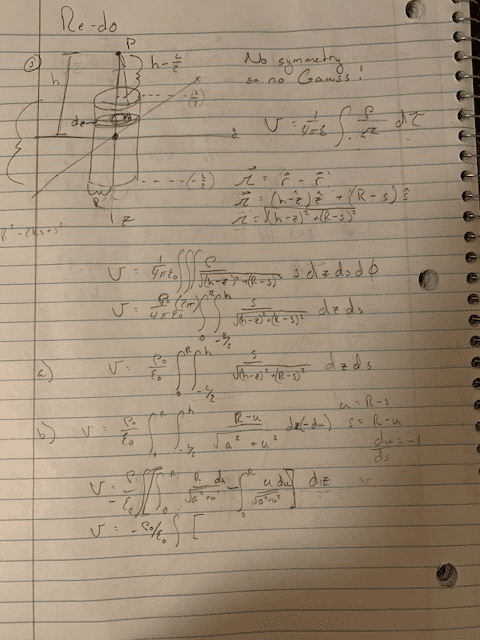# Electric Potential of point outside cylinder

jkthejetplane
Homework Statement:
So i am supposed to set up an integral with s (radial) and z to show the electric potential at a point. Part b wants you to calculate the integral in s direction and write in terms of dV/dz
Relevant Equations:
V = 1/4piEps Integral (rho/r)dTauEdit: Below is my work but i believe i have chosen the wrong values of the separation vector in the s direction. Any ideas as to what it should be?#### Attachments

Last edited:
•Delta2

Homework Helper
Gold Member
A complete statement of the problem would help us help you. What is the geometry of the charge density of the problem? Is it a thin cylinder? I guess not cause you write in your notes that there is no symmetry present. Please post a complete statement of the problem if possible.

jkthejetplane
A complete statement of the problem would help us help you. What is the geometry of the charge density of the problem? Is it a thin cylinder? I guess not cause you write in your notes that there is no symmetry present. Please post a complete statement of the problem if possible.
I edited it to put the whole original problem. thanks

Homework Helper
Gold Member
2022 Award
##(h-z)^2+(R-s)^2##? How are you defining s?

jkthejetplane
##(h-z)^2+(R-s)^2##? How are you defining s?
What do you mean? s is radial component in cylindrical

Homework Helper
Gold Member
2022 Award
What do you mean? s is radial component in cylindrical
So what is the distance from (s, Φ, z) to (0, 0, h)?

•Delta2
jkthejetplane
So what is the distance from (s, Φ, z) to (0, 0, h)?
I believe that's my main question above if i am understanding you correctly. I am not sure how to define the separation vector. Right not i have it as (h-z)zhat + (r-s)shat but i am unsure on the s direction component...

Homework Helper
Gold Member
I think the correct distance is $$|\vec{r}-\vec{r'}|=\sqrt{(h-z)^2+s^2}$$. If you do a simple figure it is straightforward pythagorean theorem.

•